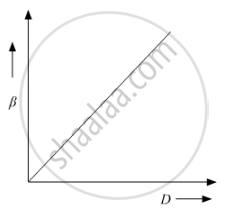# In Young'S Double Slit Experiment, Plot a Graph Showing the Variation of Fringe Width Versus the Distance of the Screen from the Plane of the Slits Keeping Other Parameters Same - Physics

In Young's double slit experiment, plot a graph showing the variation of fringe width versus the distance of the screen from the plane of the slits keeping other parameters same. What information can one obtain from the slope of the curve?

#### Solution

The fringe width in Young's double slit experiment is given by
β=λD/d/

whereλ=Wavelength of source

D=Distance between the slits and screen

d=Distance between the slits

βD

The variation of fringe width with distance of screen from the slits is given by the graph shown below:It is a linear graph with slope equal to λ/d. So, for the fringe width to vary linearly with distance of screen from the slits, the ratio of wavelength to distance between the slits should remain constant. Therefore, it is advised to take wavelengths of incident light nearly equal to the width of the slit.

Concept: Interference of Light Waves and Young’S Experiment
Is there an error in this question or solution?